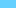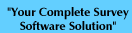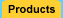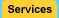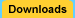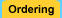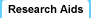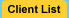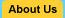Sample Size Calculator Significance Survey Design Correlation Interviewing Companies# Sample Size Calculator

This Sample Size Calculator is presented as a public service of Creative Research Systems.  You can use it to determine how many people you need to interview in order to get results that reflect the target population as precisely as needed.  You can also find the level of precision you have in an existing sample.

Before using the sample size calculator, there are two terms that you need to know. These are: confidence interval and confidence level. If you are not familiar with these terms, click here.  To learn more about the factors that affect the size of confidence intervals, click here.

This calculator requires Internet Explorer 3.0 or later or Netscape 3.0 or later or a compatible browser. Leave the Population box blank, if the population is very large or unknown.

 Confidence Level: 95% 99% Confidence Interval: Population: Sample size needed:

 Confidence Level: 95% 99% Sample Size: Population: Percentage: Confidence Interval:

## Sample Size Terminology

The confidence interval is the plus-or-minus figure usually reported in newspaper or television opinion poll results. For example, if you use a confidence interval of 4 and 47% percent of your sample picks an answer you can be "sure" that if you had asked the question of the entire relevant population between 43% (47-4) and 51% (47+4) would have picked that answer.

The confidence level tells you how sure you can be. It is expressed as a percentage and represents how often the true percentage of the population who would pick an answer lies within the confidence interval. The 95% confidence level means you can be 95% certain; the 99% confidence level means you can be 99% certain.  Most researchers use the 95% confidence level.

When you put the confidence level and the confidence interval together, you can say that you are 95% sure that the true percentage of the population is between 43% and 51%.

The wider the confidence interval you are willing to accept, the more certain you can be that the whole population answers would be within that range. For example, if you asked a sample of 1000 people in a city which brand of cola they preferred, and 60% said Brand A, you can be very certain that between 40 and 80% of all the people in the city actually do prefer that brand, but you cannot be so sure that between 59 and 61% of the people in the city prefer the brand.

## Factors that Affect Confidence Intervals

There are three factors that determine the size of the confidence interval for a given confidence level. These are: sample size, percentage and population size.

Sample Size

The larger your sample, the more sure you can be that their answers truly reflect the population. This indicates that for a given confidence level, the larger your sample size, the smaller your confidence interval. However, the relationship is not linear (i.e., doubling the sample size does not halve the confidence interval).

Percentage

Your accuracy also depends on the percentage of your sample that picks a particular answer. If 99% of your sample said "Yes" and 1% said "No" the chances of error are remote, irrespective of sample size. However, if the percentages are 51% and 49% the chances of error are much greater. It is easier to be sure of extreme answers than of middle-of-the-road ones.

When determining the sample size needed for a given level of accuracy you must use the worst case percentage (50%). You should also use this percentage if you want to determine a general level of accuracy for a sample you already have. To determine the confidence interval for a specific answer your sample has given, you can use the percentage picking that answer and get a smaller interval.

Population Size

How many people are there in the group your sample represents? This may be the number of people in a city you are studying, the number of people who buy new cars, etc. Often you may not know the exact population size. This is not a problem. The mathematics of probability proves the size of the population is irrelevant, unless the size of the sample exceeds a few percent of the total population you are examining. This means that a sample of 500 people is equally useful in examining the opinions of a state of 15,000,000 as it would a city of 100,000. For this reason, The Survey System ignores the population size when it is "large" or unknown. Population size is only likely to be a factor when you work with a relatively small and known group of people (e.g., the members of an association).

The confidence interval calculations assume you have a genuine random sample of  the relevant population.  If your sample is not truly random, you cannot rely on the intervals. Non-random samples usually result from some flaw in the sampling procedure. An example of such a flaw is to only call people during the day, and miss almost everyone who works. For most purposes, the non-working population cannot be assumed to accurately represent the entire (working and non-working) population.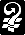Copyright © 2003  Creative Research Systems  (707) 765-1001  info@surveysystem.com